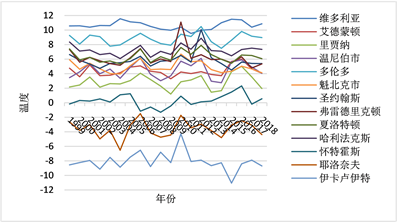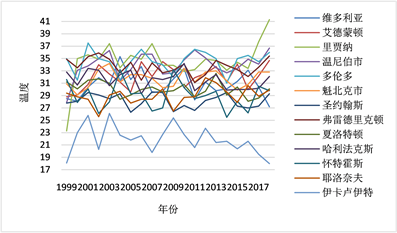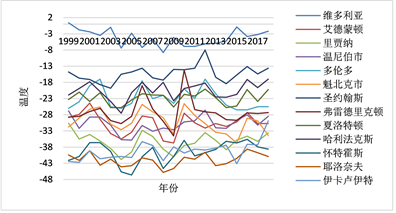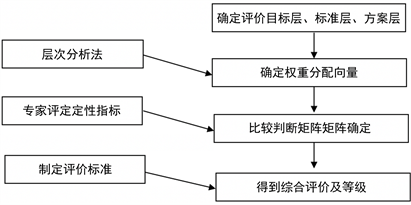﻿ 基于全球变暖对气候预测的研究

# 基于全球变暖对气候预测的研究Researching on Climate Prediction with Global Warming

Abstract: The current situation of global climate is grim, and global warming is the focus of many countries. In order to control global warming, it is more important to control from the source while remedying the current crisis. We can also find a lot from the news because of the impact of global warming on the climate. Based on the data of Canada, this paper explains the temporal and spatial variation of temperature and the frequency of extreme weather in recent decades. Considering many factors, a simple climate prediction model is established by combining AHP with entropy method. In addition, the climate data of Canada in recent ten years are analyzed, and the extreme weather model is established to judge whether the climate change is related to the extreme weather. In the context of global warming, this paper explains why extreme weather occurs frequently, and whether extreme cold weather contradicts global warming. And through the collected data and the constructed model, we can predict the climate change in the next 25 years, so that more people can understand the trend of global climate change, so as to alert people to join the team of global climate governance.

1. 引言

Xu等(2018)描述了全球变暖的速度比预计速度要快的三条证据，以及对于减缓全球变暖速度提出可行性意见 。David Spratt和IanDunlop (2019)，运用情景分析方法对从现在到2050年的气候变化的加速对人类造成的影响进行最有效的探索，并对于减少此类风险并维持人类文明提出一些建议 。Corinne Le Quéré等(2017)通过描述数据集和方法来量化全球碳预算的五个主要组成部分及其不确定性，并表明提供年度碳预算有两个目的 。Iselin Medhaug等(2017)，对“全球变暖停滞”这一争议进行探讨，并对造成这一现象的原因，以及这一现象产生的影响进行分析 。ZhengYu Liu (2012)，在一个统一的框架内，结合历史发展，讨论大西洋和太平洋年代际气候变化的动力学 。

2. 加拿大各地区温度的时空变化趋势

2.1. 加拿大各地天气变化的历史数据分析Figure 1. Annual average temperature of Canadian provinces from 1999 to 2019Figure 2. Annual maximum temperature of Canadian provinces from 1999 to 2019Figure 3. Annual minimum temperature of Canadian provinces from 1999 to 2019

2.2. 海洋表面温度历史数据分析

3. 未来25年气候变化预测

3.1. 影响因素

1) 温室气体

2) 太阳辐射

3) 海洋作用

4) 物体反射

5) 核污染

3.2. 评价指标体系的构建

3.3. 综合集成赋值法确定权重分配向量

${\omega }_{j}=\frac{{\left({w}_{j}\right)}^{\alpha }{\left({v}_{j}\right)}^{\beta }}{{\sum }_{j=1}^{m}{\left({w}_{j}\right)}^{\alpha }{\left({v}_{j}\right)}^{\beta }}\text{\hspace{0.17em}}\text{\hspace{0.17em}}\left(0\le \alpha ,\beta \le 1,\text{\hspace{0.17em}}\text{\hspace{0.17em}}\alpha +\beta =1\right)$ (1)Figure 4. Evaluation method model diagram

3.3.1. AHP

${a}_{ij}>0,\text{\hspace{0.17em}}\text{\hspace{0.17em}}{a}_{ji}=\frac{1}{{a}_{ij}}\text{\hspace{0.17em}}\text{\hspace{0.17em}}\left(i,j=1,2,\cdots ,n\right)$.

$A=\left({a}_{ij}\right)$ 为比较判断矩阵。其赋值本文通过专家技术咨询的方法获得见表2

${M}_{i}={\prod }_{j=1}^{n}{a}_{ij}\text{\hspace{0.17em}}\text{\hspace{0.17em}}\left(i=1,2,\cdots ,n\right)$,

$\stackrel{¯}{{W}_{i}}=\sqrt[n]{{M}_{i}}$,

${W}_{i}=\frac{\stackrel{¯}{{w}_{i}}}{{\sum }_{j=i}^{n}\text{ }\text{ }\stackrel{¯}{{w}_{j}}}$,

$W=\left[{W}_{1},{W}_{2},\cdots ,{W}_{n}\right]$ 即为所求的特征向量。Table 2. The scale of judgment matrix and its implication

${\lambda }_{\mathrm{max}}={\sum }_{i=1}^{n}\frac{\left(A{W}_{i}\right)}{n{W}_{i}}$,

$CI=\frac{{\lambda }_{\mathrm{max}}-n}{n-1}$,

$CR=\frac{CI}{RI}$,Table 3. The numerical table of RI for random consistency index

$CR<0.10$ 时，通过一致性检验即确定权重分配向量为 $\stackrel{¯}{W}={\left[\stackrel{¯}{{W}_{1}},\stackrel{¯}{{W}_{2}},\cdots ,\stackrel{¯}{{W}_{n}}\right]}^{\text{T}}$

$A=\left[\begin{array}{ccccc}1& 3& 7& 5& 3\\ 1/3& 1& 5& 3& 2\\ 1/7& 1/5& 1& 1/3& 1/2\\ 1/5& 1/3& 3& 1& 1/2\\ 1/3& 1/2& 2& 2& 1\end{array}\right]$

3.3.2. 熵值法确定权重

${p}_{ij}={x}_{ij}/{\sum }_{i=1}^{m}\text{ }\text{ }{x}_{ij}\text{\hspace{0.17em}}\text{\hspace{0.17em}}\left(j=1,2,\cdots ,n\right)$,

${e}_{j}=-k{\sum }_{i=1}^{m}\text{ }\text{ }{p}_{ij}\mathrm{ln}{p}_{ij}\text{\hspace{0.17em}}\text{\hspace{0.17em}}\left(j=1,2,\cdots ,n\right)$,

$k=1/\mathrm{ln}m$

${e}_{j}=\frac{-\left({\sum }_{i=1}^{m}\text{ }\text{ }{p}_{ij}\mathrm{ln}{p}_{ij}\right)}{\mathrm{ln}m},\text{\hspace{0.17em}}\text{\hspace{0.17em}}\left(0\le {e}_{j}\le 1\right)$,

${g}_{j}=1-{e}_{j}\text{\hspace{0.17em}}\text{\hspace{0.17em}}\left(j=1,2,\cdots ,n\right)$,

${V}_{j}={g}_{j}/{\sum }_{i=1}^{m}\text{ }\text{ }{g}_{i}\text{\hspace{0.17em}}\text{\hspace{0.17em}}\left(j=1,2,\cdots ,n\right)$,

$\omega ={\left[{\omega }_{1},{\omega }_{2},\cdots ,{\omega }_{n}\right]}^{\text{T}}$.

3.4. 全球气候现状分析Figure 5. Evaluation of primary index of climate prediction

3.5. 模型的计算

$\omega ={\left(0.4757,0.2374,0.0522,0.0964,0.1384\right)}^{\text{T}}$,

${B}_{1}=\left[\begin{array}{cccccccccccc}1& 3& 3& 7& 5& 3& 5& 7& 7& 5& 9& 9\\ 1/3& 1& 1/3& 1& 3& 3& 5& 3& 5& 5& 7& 9\\ 1/3& 3& 1& 3& 5& 5& 3& 5& 5& 7& 7& 9\\ 1/7& 1& 1/3& 1& 1& 3& 3& 5& 7& 5& 7& 9\\ 1/5& 1/3& 1/5& 1& 1& 1& 3& 3& 5& 7& 7& 9\\ 1/3& 1/3& 1/5& 1/3& 1& 1& 1& 3& 5& 5& 7& 9\\ 1/5& 1/5& 1/3& 1/3& 1/3& 1& 1& 3& 3& 5& 3& 7\\ 1/7& 1/3& 1/5& 1/5& 1/3& 1/3& 1/3& 1& 3& 5& 3& 7\\ 1/7& 1/5& 1/5& 1/7& 1/5& 1/5& 1/3& 1/3& 1& 5& 3& 5\\ 1/5& 1/5& 1/7& 1/5& 1/7& 1/5& 1/5& 1/5& 1/5& 1& 1/3& 5\\ 1/9& 1/7& 1/7& 1/7& 1/7& 1/7& 1/3& 1/3& 1/3& 3& 1& 5\\ 1/7& 1/9& 1/9& 1/9& 1/9& 1/9& 1/7& 1/7& 1/5& 1/5& 1/5& 1\end{array}\right]$,

${\omega }_{1}={\left(0.2628,0.1210,0.1853,0.1075,0.0836,0.0707,0.0537,0.0393,0.0288,0.0178,0.0196,0.0098\right)}^{\text{T}}$.

${B}_{2}=\left[\begin{array}{cccccccccccc}1& 3& 3& 5& 5& 7& 5& 5& 7& 9& 7& 9\\ 1/3& 1& 1& 3& 1/3& 5& 1& 3& 5& 7& 7& 9\\ 1/3& 1& 1& 3& 3& 5& 3& 3& 5& 7& 3& 7\\ 1/5& 1/3& 1/3& 1& 1& 3& 1& 5& 5& 3& 7& 5\\ 1/5& 3& 1/3& 1& 1& 5& 7& 5& 3& 5& 3& 7\\ 1/7& 1/5& 1/5& 1/3& 1/5& 1& 1& 5& 7& 7& 5& 9\\ 1/5& 1& 1/3& 1& 1/7& 1& 1& 3& 5& 5& 3& 7\\ 1/5& 1/3& 1/3& 1/5& 1/5& 1/5& 1/3& 1& 1& 3& 1& 5\\ 1/7& 1/5& 1/5& 1/5& 1/3& 1/7& 1/5& 1& 1& 5& 3& 5\\ 1/9& 1/7& 1/7& 1/3& 1/5& 1/7& 1/5& 1/3& 1/5& 1& 1/3& 3\\ 1/7& 1/7& 1/3& 1/7& 1/3& 1/5& 1/3& 1& 1/3& 3& 1& 5\\ 1/9& 9& 1/7& 1/5& 1/7& 1/9& 1/7& 1/5& 1/5& 1/3& 1/5& 1\end{array}\right]$,

${\omega }_{2}={\left(0.2172,0.1159,0.1269,0.0810,0.1240,0.0799,0.0696,0.0345,0.0375,0.0189,0.0321,0.0625\right)}^{\text{T}}$.

${B}_{3}=\left[\begin{array}{cccccccccccc}1& 1/3& 1/3& 1& 1/3& 1/3& 1/5& 1/3& 1/3& 1/5& 1/7& 1/9\\ 3& 1& 1& 3& 1/3& 1/3& 1& 1/5& 1/5& 1/3& 1& 1/7\\ 3& 1& 1& 3& 1/3& 1/5& 1/3& 1& 1/3& 1/5& 1& 1/7\\ 1& 1/3& 1/3& 1& 1& 1/3& 1/5& 1/7& 1/5& 1/7& 1/5& 1/7\\ 3& 3& 3& 1& 1& 3& 3& 1/3& 1& 1/3& 1/5& 1/3\\ 3& 3& 5& 3& 1/3& 1& 3& 3& 1& 1/3& 1/5& 1/7\\ 5& 1& 3& 5& 1/3& 1/3& 1& 1& 1/3& 3& 1/3& 1/3\\ 3& 5& 1& 7& 1/3& 1/3& 1& 1& 3& 1/3& 1/5& 1/7\\ 3& 5& 3& 5& 1& 1& 3& 1/3& 1& 5& 3& 3\\ 5& 3& 5& 7& 3& 3& 1/3& 3& 1/5& 1& 3& 5\\ 7& 1& 1& 5& 5& 5& 3& 5& 1/3& 1/3& 1& 3\\ 9& 7& 7& 7& 3& 7& 3& 7& 1/3& 1/5& 1/3& 1\end{array}\right]$,

${\omega }_{3}={\left(0.0165,0.0343,0.0344,0.0174,0.0678,0.0726,0.0699,0.0723,0.1633,0.1573,0.1406,0.1538\right)}^{\text{T}}$.

${B}_{4}=\left[\begin{array}{cccccccccccc}1& 1/3& 1/3& 1/5& 1/5& 1/7& 1/3& 1/5& 1/5& 1/3& 1/7& 1/3\\ 3& 1& 1/3& 3& 1& 1/3& 1/5& 1/5& 1/3& 1/5& 1/3& 1/5\\ 3& 3& 1& 3& 1/3& 1/3& 1/3& 1/5& 1/7& 1/5& 1/7& 1/9\\ 5& 1/3& 1/3& 1& 1& 1/3& 1/5& 1/3& 1/3& 1/7& 1/5& 1/3\\ 5& 1& 3& 1& 1& 1/3& 1/5& 1/3& 1/5& 1/7& 1/5& 1/7\\ 7& 3& 3& 3& 3& 1& 3& 1/5& 1/3& 1/5& 1/5& 1/7\\ 3& 5& 3& 5& 5& 1/3& 1& 1/3& 1/3& 1& 1/3& 1/5\\ 5& 5& 5& 3& 3& 5& 3& 1& 3& 1/3& 1& 1/3\\ 5& 3& 7& 3& 5& 3& 3& 1/3& 1& 3& 3& 3\\ 3& 5& 5& 7& 7& 5& 1& 3& 1/3& 1& 3& 5\\ 7& 3& 7& 5& 5& 5& 3& 1& 1/3& 1/3& 1& 5\\ 3& 5& 9& 3& 7& 7& 5& 3& 1/3& 1/5& 1/5& 1\end{array}\right]$,

${\omega }_{4}={\left(0.0172,0.0275,0.0269,0.0255,0.0283,0.0567,0.0644,0.1235,0.1672,0.1826,0.1450,0.1353\right)}^{\text{T}}$.

${B}_{5}=\left[\begin{array}{cccccccccccc}1& 1/3& 3& 5& 3& 3& 3& 5& 1/3& 1/3& 1/5& 1/3\\ 3& 1& 3& 1& 1/3& 3& 1/5& 1/3& 1/7& 1/3& 1/7& 1/5\\ 1/3& 1/3& 1& 5& 1& 1/3& 1/3& 1& 1/5& 1/3& 1/5& 1/3\\ 1/5& 1& 1/5& 1& 1/5& 1/3& 1/3& 1/5& 1/7& 1/5& 1/9& 1/7\\ 1/3& 3& 1& 5& 1& 5& 3& 3& 1/5& 1/3& 1/7& 1/5\\ 1/3& 1/3& 3& 3& 1/5& 1& 3& 5& 1/3& 1& 1/5& 1/3\\ 1/3& 5& 3& 3& 1/3& 1/3& 1& 1& 1/5& 1/3& 1/7& 1/3\\ 1/5& 3& 1& 5& 1/3& 1/5& 1& 1& 1/3& 1/3& 1/5& 1/3\\ 3& 7& 5& 7& 5& 3& 5& 3& 1& 5& 1/5& 1/3\\ 3& 3& 3& 5& 3& 1& 3& 3& 1/5& 1& 1/3& 1\\ 5& 7& 5& 9& 7& 5& 7& 5& 5& 3& 1& 1/5\\ 3& 5& 3& 7& 5& 3& 3& 3& 3& 1& 5& 1\end{array}\right]$,

${\omega }_{5}={\left(0.0698,0.0436,0.0284,0.0143,0.0608,0.0509,0.0419,0.0354,0.1455,0.0885,0.2173,0.2035\right)}^{\text{T}}$.Table 4. Results of hierarchical sorting

4. 全球变暖与极寒天气的关系

4.1. 影响因子的选取

“极寒天气”的出现也离不开当年大气环流背景。年积温是反映大气环流特征的一个重要表现。所以选择因子首先从影响年积温高低的因子着手。经过因子的组合分析选取8个因子：

X1：今年1月平均温度；X2：上年11月到今年2月的积温(即11~2月的月平均温度之和)；

X3：上年12月平均温度；X4：上年3月到今年2月年积温；X5：上年3~6月降水量；

X6：上年7~10月降水量；X7：上年11月~今年2月降雪量；X8：上年3~9月积温；

1) 主要因子 ${X}_{1}$ 与其条件因子的关系。当年1月平均温度 ${X}_{1}$ 满足 ${X}_{1}\le -18.5$ ( $\stackrel{¯}{{X}_{1}}=-15.5$ )且上年年积温 ${X}_{4}\ge 52.9$ ( $\stackrel{¯}{{X}_{4}}=35.6$ )，上年3~6月降水量 ${X}_{5}\le 382.1$ ( $\stackrel{¯}{{X}_{5}}=278.8$ )和上年11月~今年2月降雪量 ${X}_{7}\le 3164.9$ ( $\stackrel{¯}{{X}_{7}}=3164.9$ )，则当年积温T要下降，它等于上年积温 ${X}_{4}$ 减去负增量 $\Delta {X}_{4}$$T={X}_{4}-\Delta {X}_{4}$，因而在年积温的下降过程中要影响下一年出现“极寒天气”。

2) 主要因子 ${X}_{2}$ 与其条件因子的关系。上年11月到今年2月的积温 ${X}_{2}$ 满足 ${X}_{2}\ge \stackrel{¯}{{X}_{2}}+{\sigma }_{2}=-33$ 且上年3~6月降水量 ${X}_{5}\le 382.1$ 和今年1月平均温度 ${X}_{1}\le -12.5$ ( $\stackrel{¯}{{X}_{1}}=-15.5$ )，则上年11月到今年2月的积温为前年11月到上年2月的积温 ${X}_{2}$ 减去负增量 $\Delta {X}_{2}$ 即为 ${X}_{2}-\Delta {X}_{2}$，因而在它的下降中也要影响下一年出现“极寒现象”。

3) 主要因子 ${X}_{3}$ 与其条件因子的关系。上年12月平均温度 ${X}_{3}$ 满足 ${X}_{3}\le -16$ ( $\stackrel{¯}{{X}_{3}}=-11.9$ )，上年3~9月积温 ${X}_{8}$ 满足 ${X}_{8}\le 82$ ( $\stackrel{¯}{{X}_{8}}=74.1$ )且上年11月~今年2月降雪量 ${X}_{7}\le 3164.9$ (上年3~6月降水量 ${X}_{5}\le 382.1$ 或上年7~10月降水量 ${X}_{6}\le 358.7$ )则要影响下一年出现“极寒天气”。

4.2. 影响因子的判别式

${{X}^{\prime }}_{1}=\left\{\begin{array}{cc}1,& {X}_{1}\le -18.5\\ 0,& {X}_{1}>-18.5\end{array}$, ${{X}^{\prime }}_{2}=\left\{\begin{array}{cc}1,& {X}_{2}\ge -33\\ 0,& {X}_{2}<-33\end{array}$, ${{X}^{\prime }}_{3}=\left\{\begin{array}{cc}1,& {X}_{3}\le -16\\ 0,& {X}_{3}>-16\end{array}$,

${{X}^{\prime }}_{4}=\left\{\begin{array}{ll}0,\hfill & {X}_{4}\le 18.3\hfill \\ {\left[1+\frac{{\left({X}_{4}-18.3\right)}^{-1}}{5}\right]}^{-1},\hfill & {X}_{4}>18.3\hfill \end{array}$, ${{X}^{\prime }}_{5}=\left\{\begin{array}{ll}0,\hfill & {X}_{5}\ge 382.1\hfill \\ {\left[1+\frac{{\left(382.1-{X}_{5}\right)}^{-1}}{2}\right]}^{-1},\hfill & {X}_{5}<382.1\hfill \end{array}$,

${{X}^{\prime }}_{6}=\left\{\begin{array}{ll}0,\hfill & {X}_{6}\ge 358.7\hfill \\ {\left[1+\frac{{\left(358.7-{X}_{6}\right)}^{-1}}{2}\right]}^{-1},\hfill & {X}_{6}<358.7\hfill \end{array}$, ${{X}^{\prime }}_{7}=\left\{\begin{array}{ll}0,\hfill & {X}_{7}\ge 3164.9\hfill \\ {\left[1+\frac{{\left(3164.9-{X}_{7}\right)}^{-1}}{2}\right]}^{-1},\hfill & {X}_{7}<3164.9\hfill \end{array}$,

${{X}^{\prime }}_{8}=\left\{\begin{array}{ll}0,\hfill & {X}_{8}\ge 82\hfill \\ {\left[1+\frac{{\left(82-{X}_{8}\right)}^{-1}}{10}\right]}^{-1},\hfill & {X}_{8}<82\hfill \end{array}$, ${{X}^{″}}_{1}=\left\{\begin{array}{ll}0,\hfill & {X}_{1}\ge -12.5\hfill \\ {\left[1+\frac{{\left(-12.5-{X}_{1}\right)}^{-1}}{20}\right]}^{-1},\hfill & {X}_{1}<-12.5\hfill \end{array}$.

${P}_{a}={{X}^{\prime }}_{1}\wedge {X}_{4}^{1}\wedge {{X}^{\prime }}_{5}\wedge {{X}^{\prime }}_{7}=\left\{\begin{array}{cc}\ge 0.9时& 出现寒冬\\ <0.9时& 无寒冬出现\end{array}$

${P}_{b}={{X}^{\prime }}_{2}\wedge {{X}^{\prime }}_{5}\wedge {{X}^{″}}_{1}=\left\{\begin{array}{cc}\ge 0.9时& 出现寒冬\\ <0.9时& 无寒冬出现\end{array}$

${P}_{c}={{X}^{\prime }}_{3}\wedge {X}_{8}^{1}\wedge {{X}^{\prime }}_{7}\wedge \left({{X}^{\prime }}_{5}\vee {{X}^{\prime }}_{6}\right)=\left\{\begin{array}{cc}\ge 0.9时& 出现寒冬\\ <0.9时& 无寒冬出现\end{array}$

$P={P}_{a}\vee {P}_{b}\vee {P}_{c}=\left\{\begin{array}{cc}\ge 0.9时& 出现寒冬\\ <0.9时& 无寒冬出现\end{array}$

4.3. 极端天气模型的检验Table 5. The numerical values of forecast objects and influence factors over the years and their discrimination results

Table 6. The numerical values of forecast objects and influence factors over the years and their discrimination resultsTable 7. Average value of each influence factorTable 8. Standard deviation of each influence factor

5. 总结

 Quere, C., Andrew, R., et al. (2018) Global Carbon Budget 2017. Earth System Science Data, 10, 405-448.
https://doi.org/10.5194/essd-10-405-2018

 Medhaug, I., Stolpe, M.B., Fischer, E.M. and Knutti, R. (2017) Reconciling Controversies about the ‘Global Warming Hiatus’. Nature, 545, 41-47.
https://doi.org/10.1038/nature22315

 Meehl, G.A., Hu, A., Santer, B.D. and Xie, S. (2016) Contribution of the Interdecadal Pacific Oscillation to Twentieth-Century Global Surface Temperature Tends. Nature Climate Change, 6, 1005-1008.
https://doi.org/10.1038/nclimate3107

 Chen, X. and Tung, K.K. (2018) Global Surface Warming Enhanced by Weak Atlantic Overturning Circulation. Nature, 559, 387-391.
https://doi.org/10.1038/s41586-018-0320-y

 Xu, Y., Ramanathan, V. and Victor, D.G. (2018) Global Warming Will Happen Faster Than We Think. Nature, 564, 30-32.
https://doi.org/10.1038/d41586-018-07586-5

 Spratt, D. and Dunlop, I. (2019) Existential Climate-Related Security Risk: A Scenario Approach. Policy Paper from Breakthrough-National Centre for Climate Restoration.

 Smith, T.M. and Reynolds, R.W. (1998) A High-Resolution Global Sea Surface Temperature Climatology for the 1961-90 Base Period. Journal of Climate, 11, 3320-3323.
https://doi.org/10.1175/1520-0442(1998)011<3320:AHRGSS>2.0.CO;2

 邱欢. 140年来东海及毗邻的西北太平洋海水温度变化的特征分析[D]: [硕士学位论文]. 上海: 上海师范大学, 2017.

 Quéré, C.L., Andrew, R.M., Friedlingstein, P., et al. (2015) Global Carbon Budget 2017. Earth System Science Data Discussions, 7, 521-610.

 Liu, Z. (2012) Dynamics of Interdecadal Climate Variability: A Historical Perspective. Journal of Climate, 25, 1963-1995.
https://doi.org/10.1175/2011JCLI3980.1

 Drosdowsky, W. and Chambers, L.E. (2001) NOTES AND CORRESPONDENCE: Near-Global Sea Surface Temperature Anomalies as Predictors of Australian Seasonal Rainfall. Journal of Climate, 14, 1677-1687.
https://doi.org/10.1175/1520-0442(2001)014<1677:NACNGS>2.0.CO;2

 Edward Bryant. 气候过程和气候变化[M]. 刘东生, 等, 编译. 北京: 科学出版社, 2004: 27-28.

 周洪祥. 灾害性天气的预测方法[M]. 北京: 气象出版社, 2002: 155-160.

Top